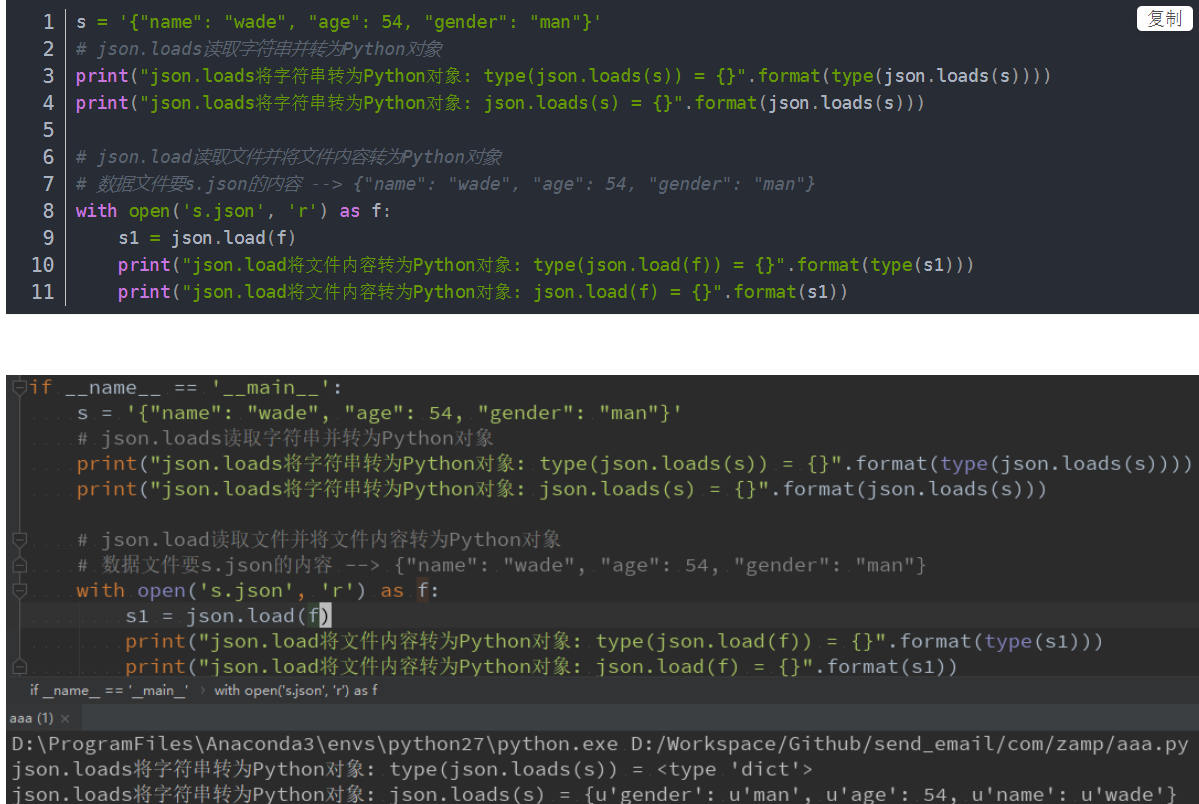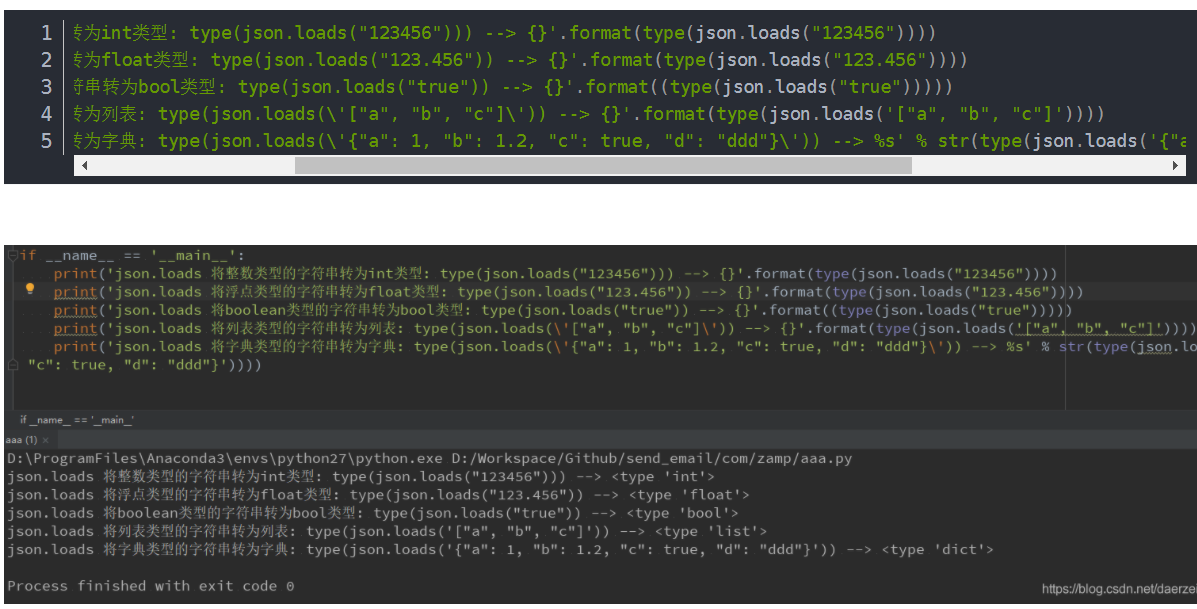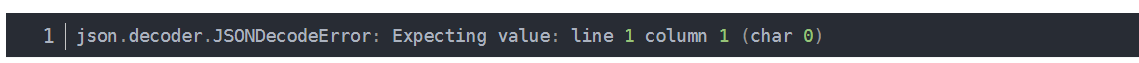# python-知识讲解-json模块的load/loads方法实战及参数详解

1. loads方法与load方法的异同

json.dumps
json.dump
json.loads
json.load

Python对象包括：

1.1不相同点：
loads操作的是字符串
load操作的是文件流
1.2 相同点

1.3 例子s = ‘{"name": "wade", "age": 54, "gender": "man"}‘
# json.loads读取字符串并转为Python对象
print("json.loads将字符串转为Python对象: type(json.loads(s)) = {}".format(type(json.loads(s))))
print("json.loads将字符串转为Python对象: json.loads(s) = {}".format(json.loads(s)))

# json.load读取文件并将文件内容转为Python对象
# 数据文件要s.json的内容 --> {"name": "wade", "age": 54, "gender": "man"}
with open(‘s.json‘, ‘r‘) as f:
s1 = json.load(f)
print("json.load将文件内容转为Python对象: type(json.load(f)) = {}".format(type(s1)))
print("json.load将文件内容转为Python对象: json.load(f) = {}".format(s1))
1
2
3
4
5
6
7
8
9
10
11

2. 转换成Python对象print(‘json.loads 将整数类型的字符串转为int类型: type(json.loads("123456"))) --> {}‘.format(type(json.loads("123456"))))
print(‘json.loads 将浮点类型的字符串转为float类型: type(json.loads("123.456")) --> {}‘.format(type(json.loads("123.456"))))
print(‘json.loads 将boolean类型的字符串转为bool类型: type(json.loads("true")) --> {}‘.format((type(json.loads("true")))))
print(‘json.loads 将列表类型的字符串转为列表: type(json.loads(\‘["a", "b", "c"]\‘)) --> {}‘.format(type(json.loads(‘["a", "b", "c"]‘))))
print(‘json.loads 将字典类型的字符串转为字典: type(json.loads(\‘{"a": 1, "b": 1.2, "c": true, "d": "ddd"}\‘)) --> %s‘ % str(type(json.loads(‘{"a": 1, "b": 1.2, "c": true, "d": "ddd"}‘))))
1
2
3
4
5
json模块会根据你的字符串自动转为最符合的数据类型，json.decoder.JSONDecodeError: Expecting value: line 1 column 1 (char 0)
————————————————

python-知识讲解-json模块的load/loads方法实战及参数详解

(0)
(0)© 2014 mamicode.com 版权所有